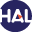Home page > 05. Volume 3 N°1 (2006) > FV schemes for a non linear Hyperbolic CL with a flux function involving (...)

# FV schemes for a non linear Hyperbolic CL with a flux function involving discontinuous coefficients

Wednesday 6 December 2006,

Full Title: Finite volume schemes for a non linear hyperbolic conservation law with a flux function involving discontinuous coefficients

Abstract:

A model for two phase flow in porous media with distinct permeabilities leads to a non linear hyperbolic conservation law with a discontinuous flux function. In this paper for such a problem, the notion of entropy solution is presented and existence and convergence of a finite volume scheme are proved. No hypothesis of convexity or genuine non linearity on the flux function is assumed, which is a new point in comparison with preceding works. As the trace of the solution along the line of discontinuity of the flux function can not be considered, this problem is more complex. To illustrate these results, some numerical tests are presented.

Paper presented by Prof. F. BenkhaldounVoir sur HAL : https://hal.archives-ouvertes.fr/hal-01114200